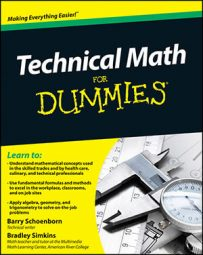##### Technical Math For DummiesGrasping some technical math basics can simplify everyday situations faced by many professionals and even non-professionals, including having to solve word problems, calculate tips, make change, or match American and metric measurements.

## Simple steps for solving word problems

Word problems aren’t just on school tests. You solve word problems every day in your work or even while you’re just out and about. Don’t worry — these steps make solving word problems easier than you think. Just follow these easy steps to take away the mystery:

2. Identify and list the facts.

Look at all the information given in the story problem and make a list of what you know.

3. Figure out exactly what the problem is asking for.

Know what you’re trying to find. The problem often states the required answer, but sometimes you have to ferret it out from the information given. If an important fact isn’t there, you can often convert some piece of the given information.

4. Eliminate excess information.

The problem may include facts that don’t help you find the solution, so clear them out of the way.

5. Pay attention to units of measurement.

For example, if dimensions are given in inches, but the answer must be in square feet, you need to convert units. You can use a table of conversions, an online calculator, or your own memory to get the conversion factors.

6. Draw a diagram.

Sometimes a diagram helps you visualize the problem (but not always).

7. Find or develop a formula.

When you see the math that needs to be done, you probably know a common math formula to use for the computation. You will find that you use some special formulas again and again.

8. Consult a reference.

If you’re stuck, look for a reference of some kind, such as a conversion chart or even a blog where someone has encountered the same problem you’re having (although as always, consider Internet sources carefully).

After you have an answer, be sure to test it. If the result is outrageously high or low, verify whether you made a mistake.

## Calculating a standard 20-percent tip

Calculating a 20-percent tip in your head may seem tricky, but here’s a, well, tip for those situations when you need to calculate that standard tip amount. And it works 100 percent of the time. In order to determine a 20-percent tip, follow these steps:

Just move the decimal point one place to the left. For a \$50.00 dinner, that’s \$5.00.

2. Double the amount created in Step 1.

10 percent x 2 = 20 percent! In this case, it’s \$5.00 x 2, which equals \$10.00.

Many cellphones and smartphones have tip calculators, but the steps provided here can help you out in a pinch if you don’t have access to your phone for some reason.

## Counting change for customers the old-fashioned way

Although many cashiers simply dump all your change in your hand at once, counting change the old-fashioned way ensures that customers get the proper amount. Here’s an easy way to make a customer’s change, using counting and simple addition:

1. Leave the payment in plain sight on top of the cash drawer.

Say your customer gives you a \$10.00 bill for a \$9.56 purchase. Put it on the cash drawer while you gather the change.

2. Count the change out and give it back to the customer, starting with the smallest coins and describing what you’re giving.

For the example of \$9.56, say

“Your purchase was \$9.56, out of \$10.00. That’s \$9.56,

plus 4 cents (4 pennies) makes \$9.60,

plus 5 cents (a nickel) makes \$9.65,

plus 10 cents (a dime) makes \$9.75,

plus 25 cents (a quarter) makes \$10.00.”

## Matching American and Metric units of measurement

If you’ve ever had to match American and metric measurement units, you know direct comparisons of these unit systems can be tricky. If you need to know the metric equivalent of an inch, for example, or the American equivalent of a kilometer, use the following table to help you make a proper comparison between the two systems of measurement.

 American Length Metric Length * millimeter inch centimeter (0.4 inches) foot (12 inches) * yard (3 feet) meter (39.37 inches) rod (16.5 feet) * furlong (1/8 mile) * mile (5,280 feet) kilometer (0.6214 miles)
 American Area Metric Area square inch square centimeter (0.1550 square inches) square foot (144 square inches) square decimeter (0.1076 square feet) square yard (9 square feet) * acre (43,560 square feet) hectare (10,000 square meters, 0.01 square kilometers, 0.4047 acres) square mile (640 acres) square kilometer (0.3861 square miles)
 American Volume Metric Volume * cubic millimeter cubic inch cubic centimeter (0.0610 cubic inches) cubic foot (1,728 cubic inches) cubic meter (0.0283 cubic feet) cubic yard (27 cubic feet) cubic meter (0.7645 cubic yards)
 American Weight Metric Weight * gram grain * dram (27.3438 grains) * ounce (437.5 grains) * pound (16 ounces, 7,000 grains) * * kilogram (1,000 grams) hundredweight (100 pounds) * ton (20 hundred weight, 2,000 pounds) tonne (1,000 kilograms, 1.1023 tons) long hundredweight (112 pounds) * long ton (20 long hundredweight, 2,240 pounds) *
 American liquid volume Metric liquid volume * milliliter fluid ounce (29.5735 milliliters) * cup (8 fluid ounces) * pint (16 fluid ounces) * * liter (1,000 milliliters, 1.0567 quarts) quart (32 fluid ounces, 2 pints) * gallon (4 quarts) *
 American Temperature Metric temperature degrees Fahrenheit degrees Kelvin